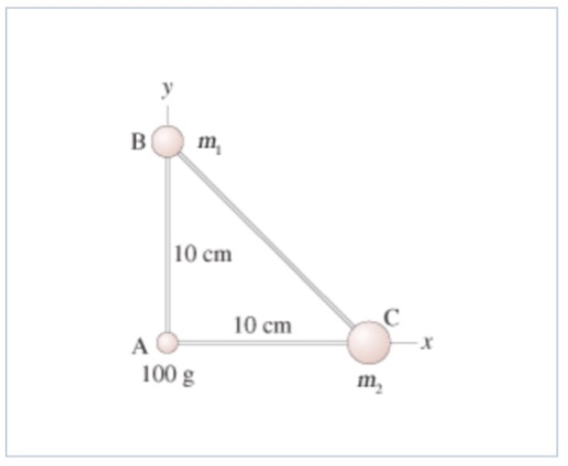# Problem: The three masses shown in the figure are connected by massless, rigid rods. Assume that m1 = 170 g and m2 = 310 g.A.) What is the x-coordinate of the center of mass?B.) What is the y-coordinate of the center of mass?

🤓 Based on our data, we think this question is relevant for Professor TBA's class at GGC.

###### FREE Expert Solution

The x-component of center of mass:

$\overline{){{\mathbf{x}}}_{\mathbf{c}\mathbf{m}}{\mathbf{=}}\frac{{\mathbf{m}}_{\mathbf{1}}{\mathbf{x}}_{\mathbf{1}}\mathbf{+}{\mathbf{m}}_{\mathbf{2}}{\mathbf{x}}_{\mathbf{2}}\mathbf{+}{\mathbf{m}}_{\mathbf{3}}{\mathbf{x}}_{\mathbf{3}}}{\mathbf{\left(}{\mathbf{m}}_{\mathbf{1}}\mathbf{+}{\mathbf{m}}_{\mathbf{2}}\mathbf{+}{\mathbf{m}}_{\mathbf{3}}\mathbf{\right)}}}$

Similarly, y-component of the center of mass:

$\overline{){{\mathbf{y}}}_{{\mathbf{cm}}}{\mathbf{=}}\frac{{\mathbf{m}}_{\mathbf{1}}{\mathbf{y}}_{\mathbf{1}}\mathbf{+}{\mathbf{m}}_{\mathbf{2}}{\mathbf{y}}_{\mathbf{2}}\mathbf{+}{\mathbf{m}}_{\mathbf{3}}{\mathbf{y}}_{\mathbf{3}}}{\mathbf{\left(}{\mathbf{m}}_{\mathbf{1}}\mathbf{+}{\mathbf{m}}_{\mathbf{2}}\mathbf{+}{\mathbf{m}}_{\mathbf{3}}\mathbf{\right)}}}$###### Problem Details

The three masses shown in the figure are connected by massless, rigid rods. Assume that m1 = 170 g and m2 = 310 g.A.) What is the x-coordinate of the center of mass?

B.) What is the y-coordinate of the center of mass?